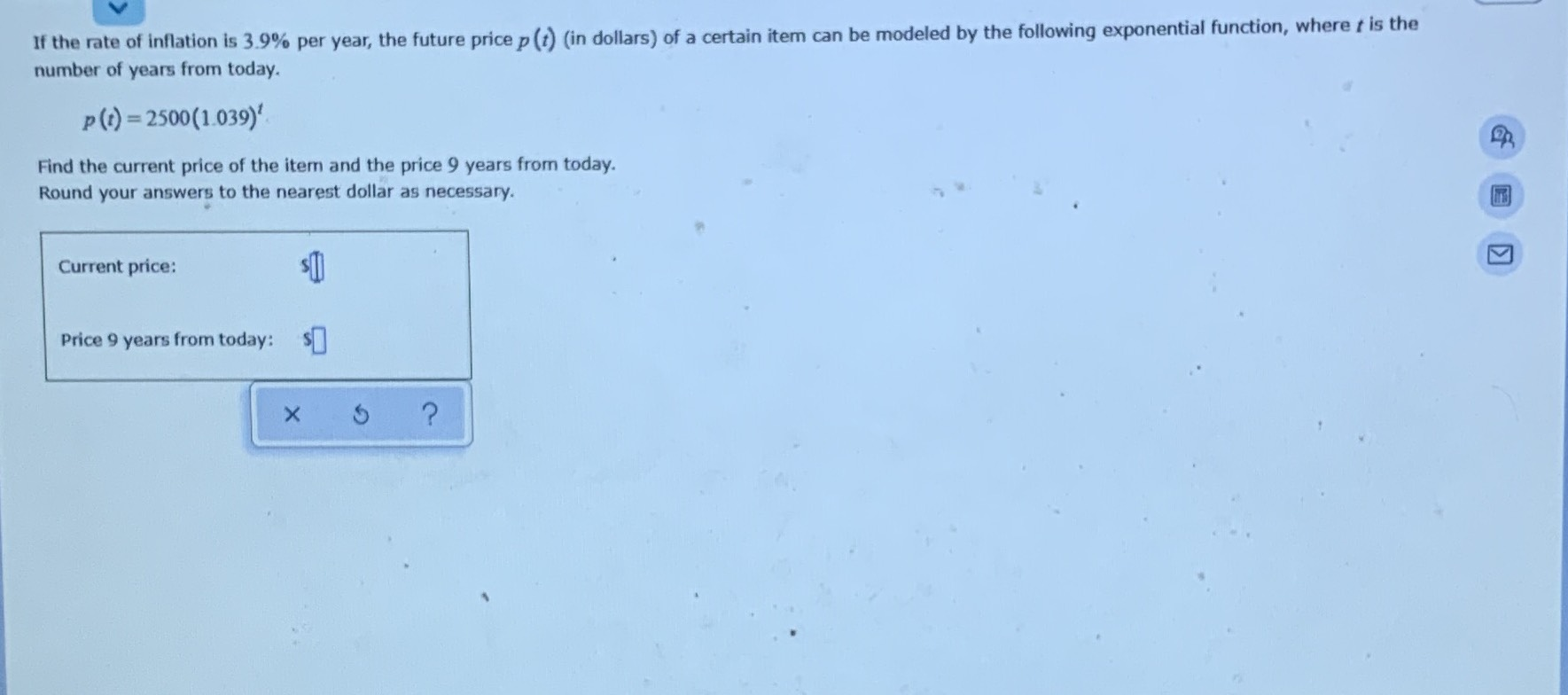### Still have math questions?

Algebra
QuestionIf the rate of inflation is $$3.9 \%$$ per year, the future price $$p ( t )$$ (in dollars) of a certain item can be modeled by the following exponential function, where $$t$$ is the number of years from today.

$$p ( t ) = 2500 ( 1.039 ) ^ { t }$$

Find the current price of the item and the price $$9$$ years from today. Round your answers to the nearest dollar as necessary.

Current price:

Price $$9$$ years from today:

$$2500$$
$$3528$$